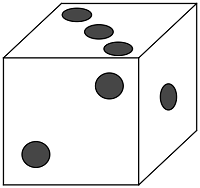# SAT Solid Geometry

Geometry Level 1A white die has gray dots. If the area of each of the die's faces is $a$ and the area of each of the die's dots is $b,$ what is the area of the white surface on the die in terms of $a$ and $b?$

(A) $\ \ 4a-21b$
(B) $\ \ 6a - 6b$
(C) $\ \ 6a-21b$
(D) $\ \ 4a^2 - 21\pi b$
(E) $\ \ 6a^2 - 21\pi b^2$

×

Problem Loading...

Note Loading...

Set Loading...